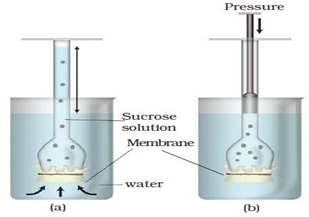Apply concept of water potential and osmosisThe pressure applied in this experiment is

1. Hydrostatic Pressure
2. Colloidal pressure
3. Osmotic pressure
4. Pressure potential
High Yielding Test Series + Question Bank - NEET 2020

Difficulty Level:

Osmotic pressure is a function of

1. Osmotic potential
2. Solute concentration
3. Solvent amount
4. Water potential
High Yielding Test Series + Question Bank - NEET 2020

Difficulty Level:

Osmotic pressure is the

1. Pressure required to stop the water diffusing in
2. Function of solute concentration
3. More the solute, more is the pressure needed to stop endosmosis
4. All of these
High Yielding Test Series + Question Bank - NEET 2020

Difficulty Level:

Numerically osmotic pressure is equal to  the

1. Osmotic potential
2. Solute potential
3. Both A and B but sign is opposite
4. Pressure potential
High Yielding Test Series + Question Bank - NEET 2020

Difficulty Level:

Isotonic solution is

1. A solution which is more concentrated than the cytoplasm
2. A solution which is more dilute than the cytoplasm
3. A solution which balances the osmotic pressure of the cytoplasm
4. A solution which is less dilute than the cytoplasm
High Yielding Test Series + Question Bank - NEET 2020

Difficulty Level:

Hypotonic solution

1. solution which is more concentrated than the cytoplasm
2. A solution which is more dilute than the cytoplasm
3. A solution which balances the osmotic pressure of the cytoplasm
4. A solution which is less dilute than the cytoplasm
High Yielding Test Series + Question Bank - NEET 2020

Difficulty Level:

Hypertonic solution

1. solution which is more concentrated than the cytoplasm
2. A solution which is more dilute than the cytoplasm
3. A solution which balances the osmotic pressure of the cytoplasm
4. A solution which is equal to that of cytoplasm in terms of concentration
High Yielding Test Series + Question Bank - NEET 2020

Difficulty Level:

Plasmolysis

Which one is incorrect.

1. External solution is hypertonic
2. Water moves out of the cell
3. Cell membrane shrinks away from it's cell wall
4. Firstly cell sap comes out then cytoplasmic water.
High Yielding Test Series + Question Bank - NEET 2020

Difficulty Level:

What occupies the space between the cell wall and the shrunken protoplast in the plasmolysed cell?

1. Hypertonic solution
2. Hypotonic solution
3. Water
4. Cytoplasmic content
High Yielding Test Series + Question Bank - NEET 2020

Difficulty Level:

When water goes into the cell and out of the cell are in equilibrium, then the solution and cell are respectively

1. Isotonic, Flaccid
2. Hypotonic, Flaccid
3. Hypertonic, Turgid
4. Hypotonic, Turgid